Courses

# Test: Meaning Of Derivatives

## 10 Questions MCQ Test Mathematics For JEE | Test: Meaning Of Derivatives

Description
This mock test of Test: Meaning Of Derivatives for JEE helps you for every JEE entrance exam. This contains 10 Multiple Choice Questions for JEE Test: Meaning Of Derivatives (mcq) to study with solutions a complete question bank. The solved questions answers in this Test: Meaning Of Derivatives quiz give you a good mix of easy questions and tough questions. JEE students definitely take this Test: Meaning Of Derivatives exercise for a better result in the exam. You can find other Test: Meaning Of Derivatives extra questions, long questions & short questions for JEE on EduRev as well by searching above.
QUESTION: 1

###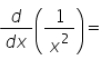Solution:

d/dx(1/x2 ) = -2 (x -2-1) = -2* (x-3) = -2/x3

QUESTION: 2

### The derivative of f(x) = 99x at x = 100 is:​

Solution:

f'(100) = lim(h → 0)  [f(100+h) - f(100)]/h
= lim(h → 0) [99(100+h) - 99(100)]/h
= lim(h → 0) [9900 + 99h - 9900]/h
= lim(h → 0) 99h/h
= lim(h → 0)  99
= 99

QUESTION: 3

### Find the derivative of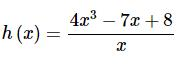Solution:

We’ll first need to divide the function out and simplify before we take the derivative. Here is the rewritten function.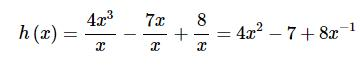The derivative is,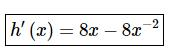QUESTION: 4

Derivative of sum of two functions is …… of the derivatives of the functions.​

Solution: In calculus, the sum rule in differentiation is a method of finding the derivative of a function that is the sum of two other functions for which derivatives exist. This is a part of the linearity of differentiation.
QUESTION: 5

The derivative of the constant function f(x) = a for a fixed real number ‘a’ is:​

Solution:

Let f(x)=a , where a is a fixed real number.
So, f(x+h)=a
So, d/dx(f(x))=limh→0f[(x+h)−f(x)]/h
=limh→0 (a−a)/h
= 0
Hence, d/dx(a) = 0 , where a is a fixed real number.

QUESTION: 6

Derivative of sum of two functions is sum of the derivatives of the functions. If , f and g be two functions such that their derivatives are defined in ______.​

Solution:

The process of determining the derivative of a function is known as differentiation. It is clearly visible that the basic concept of derivative of a function is closely intertwined with limits. Therefore, it can be expected that the rules of derivatives are similar to that of limits. The following rules are a part of algebra of derivatives:

Consider f and g to be two real valued functions such that the differentiation of these functions is defined in a common domain.

QUESTION: 7

The derivative of f(x) = 1/x3

Solution:
QUESTION: 8

Derivative of quotient of two functions f(x) and g(x); g(x) ≠ 0 is given by  is given by

Solution:

This is a formula of question.

QUESTION: 9

If f is a real valued function and c is a point in its domain, then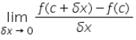is ;

Solution:

This is a formula for finding derivative or differentiation which is represented by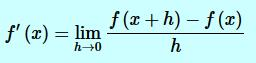but here at the place of x , c is written So this is equal to f'(c)

QUESTION: 10

The derivative at x = 2/3 of the function f(x) = 3x is:​

Solution:

f(x) = 3x
f’(x) = lim(h→0) [f(x+h) - f(x)]/h
= f(x+h) = 3(x+h)
f’(x) = = lim(h→0) [3(x+h) - 3(x)]/h
Putting x = ⅔
f’(⅔) = lim(h→0) [3(⅔+h) - 3(⅔)]/h
= lim(h→0) [(6+3h)-6]/h
=lim(h→0) 3h/h
lim(h→0) 3
The derivative of the function f’(⅔) = 3